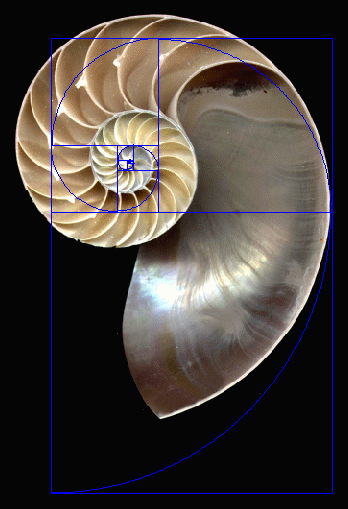#### 12/05/2017

BEAUTIFUL EQUATIONS

 Most people learn and memorize mathematical equations for a class, then forget them as soon as they walk out of the door. However, to some, mathematical equations can be viewed as an art. Given here are 10 fascinating and mind bending equations.  See  www.legitscoop.com/10-mind-blowing-mathematical-equations/#prettyPhoto and http://epicmath.org/equations/   1. Euler’s Identity$e^{i\pi} + 1 = 0$ e is Euler's number, the base of natural logarithms, i is the imaginary unit, which satisfies i2 = −1, and π is pi, the ratio of the circumference of a circle to its diameter. This is a famous equation relating the random values of  e, pi and the square root of -1. For some people, this is considered the most beautiful equation in mathematics. A more general formula is when the value is -1, which is 0, resulting in Euler’s identity as -1                 2. The Euler Product Formula$\sum_{n=1}^\infty\frac{1}{n^s} = \prod_{p \text{ prime}} \frac{1}{1-p^{-s}}$          The left symbol is an infinite sum, and on the right is the infinite product. This equation relates the natural numbers (n = 1, 2, 3, 4, 5, ) on the left side to the prime numbers (p = 2, 3, 5, 7, 11,) on the right side. You  can choose “s” to be any number greater than 1, and the equation will be true. The left side commonly represents the Riemann Zeta function.   3. The Gaussian Integral$\displaystyle\int_{-\infty}^\infty e^{-x^2}dx = \sqrt{\pi}$          The function is quite ugly to integrate. However, when it is carried out across the entire real line, which is minus infinity to infinity, it gives a strangely clean answer. When you look at it for the fist time, it is not clear that the area below the curve is the square root of pi. In statistics, this formula holds high importance because it represents normal distribution.   4. The Cardinality of the Continuum${\mathbb{R}} \sim {2^{\mathbb{N}}}$             According to this equation, the cardinality of the real numbers is equal to the cardinality of all the subsets of natural numbers. Georg Cantor, the founder of set theory proved it. It is a stunning equation because it states that a continuum is not countable . Continuum Hypothesis states that there is no cardinality between its related statement . The statement has a highly bizarre property: it can’t be proved or disproved.   5. The Analytic Continuation of the Factorial$\displaystyle n! = \int_{0}^{\infty} {x^n e^{-x} \,dx}$          The commonly defined factorial function is  n! = n(n-1)(n-2)…1, but this definition only “works” as far as positive integers are concerned. The integral equation makes “Factorial” work for fractions and decimals as well (including negative numbers and complex numbers). The Gamma function is used to define the same integral for n-1.   6. The Pythagorean Theorem$a^2 + b^2 = c^2$           This is perhaps the most familiar equation of all given here. The Pythagorean theorem relates to the sides of a right triangle. The length of the legs of the triangle are “a” and “b” whereas, c is the length of the hypotenuse. This equation relates triangles to squares as well.   7. The Explicit Formula for the Fibonacci Sequence$F(n) = \frac{(\varphi)^n - (-\frac{1}{\varphi})^n}{\sqrt{5}}$                   Many people know the Fibonacci sequence (0, 1, 1, 2, 3, 5, 8, 13, 21, 34, 55… where each number is the sum of the previous two numbers). Few are familiar with the fact that there is a formula to know any given Fibonacci number. That is, to find the 100th Fibonacci number, you don’t have to calculate the first 99 numbers. You can just throw 100 into the formula. Even with all the square roots and divisions, the answer will always be an exact positive integer.   8. The Basel Problem$\displaystyle1 + \frac{1}{4}+\frac{1}{9}+\frac{1}{16}+\frac{1}{25}+\cdots =\frac{\pi^2}{6}$            Euler first proved this equation. According to this equation, when you take the reciprocal of all the square numbers, and add them together, you get pi squared over six. This sum is just the function on the left side of Equation 2 earlier in this post, “with s = 2″. That formula is the Riemann zeta function, zeta of 2 is pi squared over six.   9. The Harmonic Series$\displaystyle1 + \frac{1}{2}+\frac{1}{3}+\frac{1}{4}+\frac{1}{5}+\cdots =\infty$         This appears to be a totally counterintuitive equation because, according to it,  if a bunch of numbers that keep getting smaller (and eventually become zero) is added, they still reach infinity. When all the numbers are squared, it doesn’t add up to infinity (it adds up to pi squared over six). The harmonic series is in fact just zeta of 1.   10. Explicit Formula for the Prime Counting Function$\displaystyle\pi(x) = \sum_{n = 1}^\infty \frac{\mu(n)}{n} J(\sqrt[n]{x})$ where${J(x)}$ is defined as$\displaystyle J(x) = Li(x) + \sum_{\rho} Li(x^\rho) - \log 2 + \int_{x}^\infty \frac{dt}{t(t^2 - 1)\log t}$ Prime numbers are numbers that have no divisors other than 1 and themselves. The primes below 100 are 2, 3, 5, 7, 11, 13, 17, 19, 23, 29, 31, 37, 41, 43, 47, 53, 59, 61, 67, 71, 73, 79, 83, 89, 97. It seems that there is no apparent pattern to finding prime numbers. In some runs of numbers one gets a lot of primes, in other runs there are no primes. However, one trick to distinguishing, or at least experimenting if a number is prime or not, is to note if the last number is an odd number.

Music Math and Symmetry  See https://www.youtube.com/watch?v=V5tUM5aLHPA

 Home | Mathematics and  Beauty | Mathematics of Prime Numbers | Mathematicians | Mathematical Topics | Math Humor | Mathematics and Music | Mathematics Odds and Ends | Mathematics Series | Mathematics Interesting Mumbers